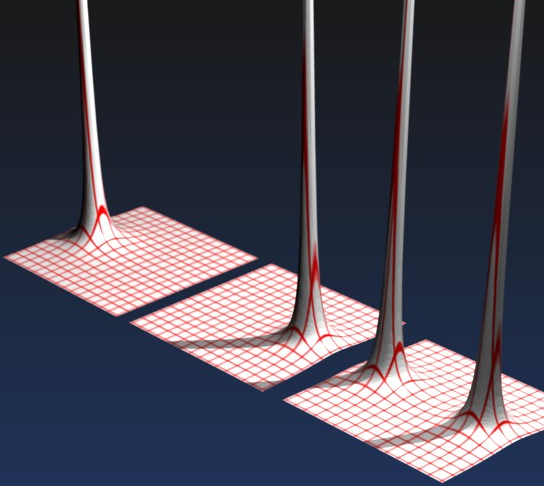Grad is short for gradient, it takes a scalar field as input and returns a vector field, for a 3 dimensional vector field it is defined as follows:

 grad(s) = i ∂s + j ∂s + k ∂s ∂x ∂y ∂z

where,

• i,j and k are unit vectors representing the axis of the Cartesian coordinates.
• s is the scalar field, i.e. a scalar value which is a function of its position.

Or in more conventional vector notation:

 ∂s ∂x
 ∂s ∂y
 ∂s ∂z

The vector returned has a direction which shows the greatest rate of change and its magnitude |v| tells us what that rate of change is. If you apply a grad to, say the height of a hill, you will get a vector pointing directly uphill, the length of which tells you how steep the hill is. Perhaps that may not be a good example as the height is normally the third dimension in a position vector but here we are separating it out as a scalar on a 2 dimensional map. Another example might be temperature, which is a scalar quantity, which might vary over 3 dimensions. grad of this temperature field would show where the temperature is changing most rapidly and would give the direction of heat flow.

If n is a normal to the contour lines then

where:

n = normal vector

If the contour lines are closer together then grad(s) will be greater.

## Vector Operator

The 'Del' operator represented by the 'Nabla' symbolis used to represent the vector operator, as follows:= i ∂ + j ∂ + k ∂ ∂x ∂y ∂z

or in more conventional vector notation:=
 ∂ ∂x
 ∂ ∂y
 ∂ ∂z

The del symbol does not have any meaning on its own, only when applied to a scalar field. Or a vector field as shown later.s = grad(s)

## Sum of Scalar Fields

In the diagram below we have plotted the potential energy (scalar) field for:

1. a single mass at x=-3
2. a single mass at x=+3
3. two masses at x=-3 and x=+3.how this plot was produced.

As we can see the field for the two masses is the sum of the other two fields.

## Identities

sum of scalar fields:

product of scalar fields:

grad of dot product of vector fields:

grad(v1v2) = (v2) v1 + (v1) v2 + v2 × curl(v1) +v1 × curl(v2)

where:

• v1, v2 = vectors
•= del operator
• • = vector dot product
• × = vector cross product
• curl() = curl function